# Area + fractions - math problems

#### Number of problems found: 82

• Living roomMarina's new living room is a rectangle with a length of 8 meters and a width of 5 meters. She wants to buy a carpet to cover half of the living room and each square meter of the carpet has a cost of \$4. How much will be the total cost for Marina?
• James 3James grows corn on 1/4 of his land. If he has 65 acres of land, how much of the land is used for growing corn?
• A triangle 2A triangle has a base of 2 inches and a height of 2 3/4 inches. What is the area of the triangle?
• MegapizzaMegapizza will be divided among 100 people. First gets 1%, 2nd 2% of the remainder, 3rd 3% of the remainder, etc. Last 100th 100% of the remainder. Which person got the biggest portion?
• Mr. PeterMr. Peter mowed 2/7 of his lawn. His son mowed 1/4 of it. Who mowed the most? How much of the lawn still need to be mowed?
• Bathroom 3De Mesa family will soon be occupying their newly renovated house . However the bathroom, measuring 10ft by 16ft, still to be covered with tiles . If the tiles that they desire measures 2/5 ft by 2/5ft, how many tiles will they need to cover the bathroom
• Jewelry box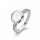The bottom of Zeyda's jewelry box is a rectangle with the length of 5 3/8 inches and a width of 3 1/4 inches. What is the area of the bottom of the jewelry box?
• Roll of wallpaperAn art student uses a roll of wallpaper to decorate two gift boxes. The student will use 3 1/3 yards of paper for one box and 5/6 yard of paper for the other box. The paper must be cut into pieces that are 1/6 yard long. How many pieces will the student c
• The gardenThe garden has the shape of an isosceles trapezoid whose bases are 64 m and 24 m long, the height is 25 m. On what area of the garden is it possible to grow vegetables if a fifth of the area is occupied by a cottage, lawn and path?
• Tree planting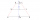The trapezoidal garden has bases 44 m and 16 m long. Their distance is 25 m. How many square meters of its area will remain for tree planting if we use 1/5 of the entire site to construct a cottage, backyard, and road. Is it possible to determine the leng
• Garden GThe rectangular, trapezoidal garden has a base length of 81m, 76m, and a vertical arm of 12m. Calculate how many m2 of the area will remain for planting greenery if 1/3 of the area is built. Calculate the consumption of mesh for land fencing.
• On the floor 2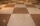The floor area of a living room is 9 7/9 m2. A carpet with an area of 5 5/8 m2 is placed on the floor. Find the area of the room that is not covered with carpet.
• Length 7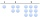Length equals 7/6 inch in width equals 7/9 inches the area is?
• GardensThe area of the square garden is 3/4 of the area of the triangular garden with sides of 80 m, 50 m, 50 m. How many meters of the fence do we need to fence a square garden?How many times does the surface of a sphere decrease if we reduce its radius twice?
• Half of halvesHalf of the square we cut off, then half of the rest, etc. Five cuts we made in this way. What part of the content of the original square is the content of the cut part?
• Cllub flag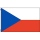The children had the club flag sewn on. The red color covered 2/20 of the total area of the flag, the blue area a fifth, the yellow 19% of the total area. What% is the green area occupied?
• Garden exchangeThe garden has a rectangular trapezoid shape, the bases of which have dimensions of 60 m and 30 m and a vertical arm of 40 m. The owner exchanged this garden for a parallelogram, which is 7/9 of the area of a trapezoidal garden. What is the size of the ne
• Triangular prismThe base of the perpendicular triangular prism is a rectangular triangle with a hypotenuse of 10 cm and one leg of 8 cm. The prism height is 75% of the perimeter of the base. Calculate the volume and surface of the prism.
• Ratio of volumesIf the heights of two cylindrical drums are in the ratio 7:8 and their base radii are in the ratio 4:3. What is the ratio of their volumes?

Do you have an exciting math question or word problem that you can't solve? Ask a question or post a math problem, and we can try to solve it.

We will send a solution to your e-mail address. Solved examples are also published here. Please enter the e-mail correctly and check whether you don't have a full mailbox.

Need help to calculate sum, simplify or multiply fractions? Try our fraction calculator. Area - math problems. Fraction Word Problems.